## March 23, 2016

### The Involute of a Cubical Parabola

#### Posted by John BaezIn his remarkable book The Theory of Singularities and its Applications, Vladimir Arnol’d claims that the symmetry group of the icosahedron is secretly lurking in the problem of finding the shortest path from one point in the plane to another while avoiding some obstacles that have smooth boundaries.

Arnol’d nicely expresses the awe mathematicians feel when they discover a phenomenon like this:

Thus the propagation of waves, on a 2-manifold with boundary, is controlled by an icosahedron hidden at an inflection point at the boundary. This icosahedron is hidden, and it is difficult to find it even if its existence is known.

I would like to understand this!

I think the easiest way for me to make progress is to solve this problem posed by Arnol’d:

Puzzle. Prove that the generic involute of a cubical parabola has a cusp of order 5/2 on the straight line tangent to the parabola at the inflection point.

There’s a lot of jargon here! Let me try to demystify it. (I don’t have the energy now to say how the symmetry group of the icosahedron gets into the picture, but it’s connected to the ‘5’ in the cusp of order 5/2.)

A cubical parabola is just a curve like $y = x^3$:It’s a silly name. I guess $y = x^3$ looked at $y = x^2$ and said “I want to be a parabola too!”

The involute of a curve is what you get by attaching one end of a taut string to that curve and tracing the path of the string’s free end as you wind the string onto that curve. For example: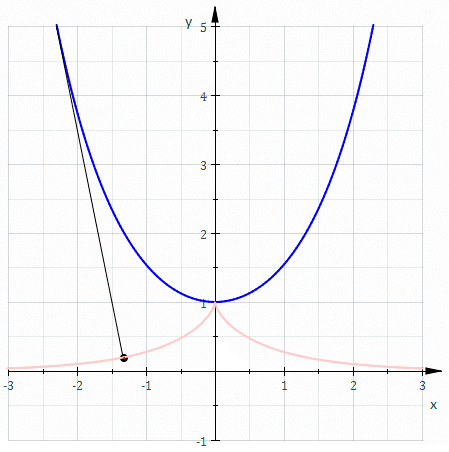Here our original curve, in blue, is a catenary: the curve formed by a hanging chain. Its involute is shown in red.

There are a couple of confusing things about this picture if you’re just starting to learn about involutes. First, Sam Derbyshire, who made this picture, cleverly moved the end of the string attached to the catenary at the instant the other end hit the catenary! That allowed him to continue the involute past the moment it hits the catenary. The result is a famous curve called a tractrix.

Second, it seems that the end of the string attached to the catenary is ‘at infinity’, very far up.

But you don’t need to play either of these tricks if you’re trying to draw an involute. Take a point $p$ on a curve $C$. Take a string of length $\ell$, nail down one end at $p$, and wind the string along $C$. Then the free end of your string traces out a curve $D$.

$D$ is called an involute of $C$. It consists of all the points you can get to from $p$ by a path of length $\ell$ that doesn’t cross $C$.

So, Arnol’d’s puzzle concerns the involute of the curve $y = x^3$.

He wants you to nail down one end of the string at any ‘generic’ location. So, don’t nail it down at $x = 0, y = 0$, since that point is different from all the rest. That point is an inflection point, where the curve $y = x^3$ switches from curving down to curving up!

He wants you to wind the string along the curve $y = x^3$, forming an involute. And he wants you to see what the involute does when it crosses the line $y = 0$.

This is a bit tricky, since the region $y \le x^3$ is not convex. If you nail your string down at $x = -1, y = -1$, your string will have to start out above the curve $y = x^3$. But when the free end of your string crosses the line $y = 0$, the story changes. Now your string will need to go below the curve $y = x^3$.

It’s a bit hard to explain this both simply and accurately, but if you imagine drawing the involute with a piece of string, I think you’ll encounter the issue I’m talking about. I hope I understand it correctly!

Anyway, suppose you succeed in drawing the involute. What should you see?

Arnol’d says the involute should have a ‘cusp of order 5/2’ somewhere on the line $y = 0$.

A cusp of order 5/2 is a singularity in an otherwise smooth curve that looks like $y^2 = x^5$ in some coordinates. In a recent post I described various kinds of cusps, and in a comment I mentioned that the cusp of order 5/2 was called a rhamphoid cusp. Strangely, I wrote all that before knowing that Arnol’d places great significance on the cusp of order 5/2 in the involute of a cubical parabola!

Simon Burton drew some nice cusps of order 5/2. The curve $y^2 = x^5$ looks like this: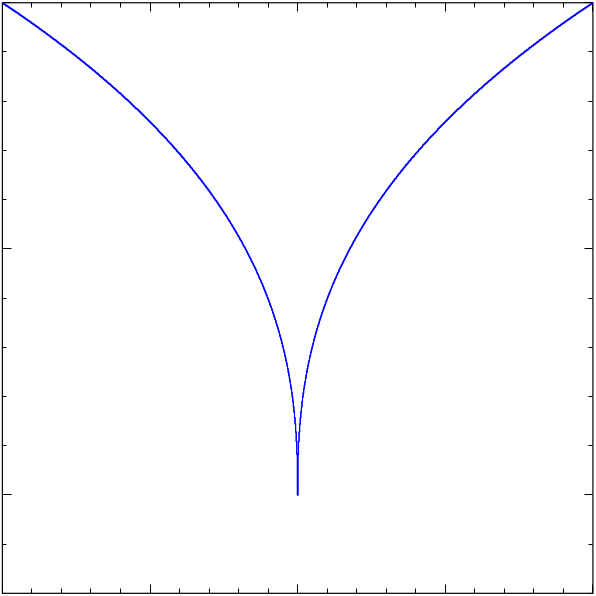This is a more typical curve with a cusp of order 5/2:

$(x-4y^2)^2 - (y+ 2x)^5 = 0$

It looks like this: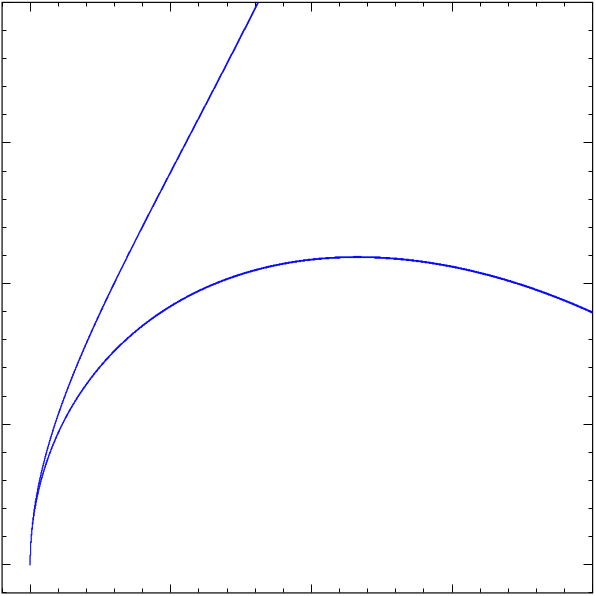It’s not as symmetrical as the curve $y^2 = x^5$, but Arnol’d emphasizes that you should usually expect this sort of shape:

It is easy to recognize this curve in experimental data, since after a generic diffeomorphism the curve consists of two branches that have equal curvatures at the common point, and hence are convex from the same side [….]

So, if we draw the involutes of a cubical parabola we should see something like this! And indeed, Marshall Hampton has made a great online program that draws these involutes. Here’s one: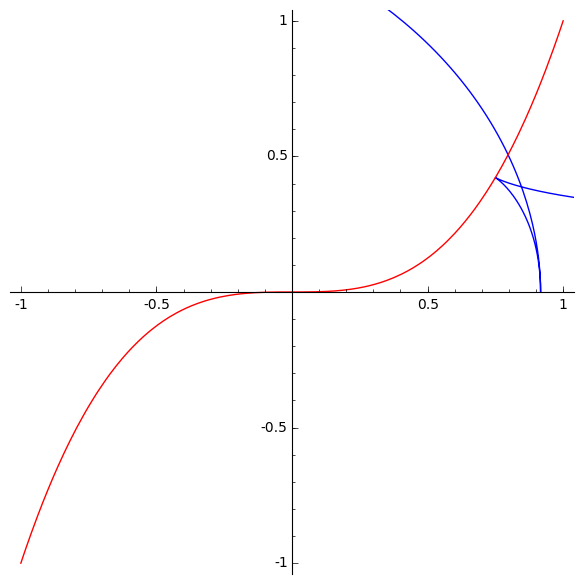The blue curve is the involute. It looks like it has a cusp of order 5/2 where it hits the line $latex y = 0.$ It also has a less pointy cusp where it hits the red curve $y = x^3.$ Like the cusp in the tractrix, this should be a cusp of order 3/2, also known as an ordinary cusp.

### Hints

Regarding the easier puzzle I posed above, Arnol’d gives this hint:

HINT. The curvature centers of both branches of the involute, which meet at the point of the inflectional tangent, lie at the inflection point, hence both branches have the same convexity (they are both concave from the side of the inflection point of the boundary).

That’s not what I’d call crystal clear! However, I now understand what he means by the two ‘branches’ of the involute. They come from how you need to change the rules of the game as the free end of your string crosses the line $y = 0$. Remember, I wrote:

If you nail your string down at $x = -1, y = -1$, your string will have to start out above the curve $y = x^3$. But when the free end of your string crosses the line $y = 0$, the story changes. Now your string will need to go below the curve $y = x^3$.

When the rules of the game change, he claims there’s a cusp of order 5/2 in the involute.

I also think I finally understand the picture that Arnol’d uses to explain what’s going on: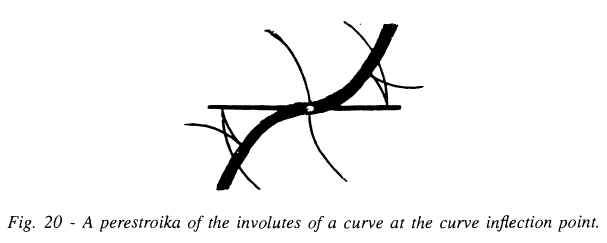It shows the curve $y = x^3$ in bold, and three involutes of this curve. One involute is not generic: it goes through the special point $x = 0, y = 0$. The other two are. They each have a cusp of order 5/2 where they hit the line $y = 0$, but also a cusp of order 3/2 where they hit the curve $y = x^3$. We can recognize the cusps of order 5/2, if we look carefully, by the fact that both branches are convex on the same side.

But again, the challenge is to prove that these involutes have cusps of order 5/2 where they hit the line $y = 0$. A cusp of order 7/2 would also have two branches that are convex on the same side!

Here’s one more hint. Wikipedia says that if we have a curve

$C :\mathbb{R} \to \mathbb{R}^2$

parametrized by arclength, so

$|C^\prime(s)|=1$

for all $s$, then its involute is the curve

$D :\mathbb{R} \to \mathbb{R}^2$

given by

$D(s) = C(s)- s C^\prime(s)$

Strictly speaking, this must be an involute. And it must somehow handle the funny situations I described, where the involute fails to be smooth. I don’t know it does this.

Posted at March 23, 2016 4:50 PM UTC

TrackBack URL for this Entry:   https://golem.ph.utexas.edu/cgi-bin/MT-3.0/dxy-tb.fcgi/2873

### Re: The Involute of a Cubical Parabola

The equation $D(s) = C(s) - s C'(s)$ is easy to understand. Imagine a string of infinite length lying on top of the curve $C$. Cut it at a point $p$. For each real number $s$, go a distance $s$ along the curve $C$ (in one direction for $s \gt 0$ and the other for $s \lt 0$) to a point we’ll call $C(s)$, and straighten the string between $p$ and the point you go to. Align the straightened string with the tangent $C'(s)$. Its endpoint will be $D(s)$.

Now $D$ is a smooth function. How does that match the singularities shown? Well, $D$ just slows down as you approach those singular points. The image of a smooth curve isn’t necessarily smooth…

Posted by: Dan Christensen on March 23, 2016 5:54 PM | Permalink | Reply to this

### Re: The Involute of a Cubical Parabola

Thanks! This formula gives one particular involute of $C(s)$. What’s the general one?

I was surprised by the appearance of a derivative in this formula, because there’s a reverse process to the involute, called the ‘evolute’, and elsewhere Jesse McKeown emphasized an analogy

involute:integral::evolute:derivative

If you don’t know what the evolute of a curve is, another of Sam Derbyshire’s pictures should explain it pretty quick. Just as the tractrix is an involute of the catenary:so the catenary is the evolute of the tractrix: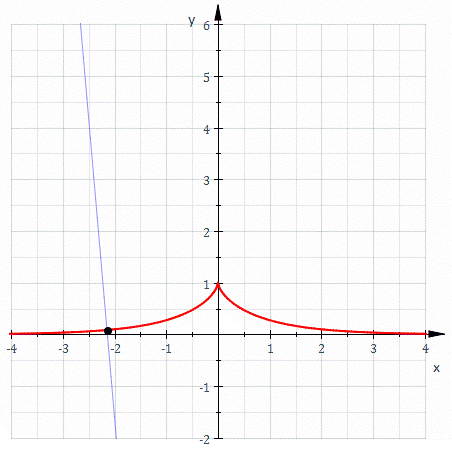A curve has one evolute but a 1-parameter family of involutes, the evolute of the involute is the original curve, etc.

Jesse wrote:

So, to a piecewise convex curve there is an envelope of tangent lines; and to another piecewise convex curve there is an envelope of normal lines; and curve B is an involute of curve A (also pronounced: A is the evolute of B) if the Tangent envelope of A is the Normal envelope of B. Curious fact: The evolute of an algebraic curve is another algebraic curve. Curiouser fact: some algebraic curves are not the evolute of any algebraic curve — an ellipse is a very good bad example. (I also think evolutes are the right way to think about the Four-Vertex Theorem)

This should sound like a similar relationship between integrals (involutes) and derivatives (evolutes), in part because constructing an involute is solving a differential equation, while constructing the evolute is basically dividing by a derivative; but more: because of the string picture described above, (distance to an) involute measures arc length. Corollary: the arclengths between algebraic points on evolutes of algebraic curves are algebraic numbers.

Curiouser and curiouser fact: Apollonius mentioned evolutes at least once, and Archimedes’ Spiral is trying very hard to be a circle involute; but the semicubic parabola, incidentally the evolute of the ordinary parabola, seems to have been the first noticed algebraic curve with piecewise algebraic arclength, around 1659. Rational hypocycloids are further examples (similar to their own evolutes!) but I don’t know who first measured them. Cf Richeson 2013, esp. section 6 (starting on p. 11).

However, none of this has much to do with finite simple Lie algebras or Coxeter groups.

Posted by: John Baez on March 23, 2016 6:52 PM | Permalink | Reply to this

### Re: The Involute of a Cubical Parabola

Dan wrote:

Now $D$ is a smooth function. How does that match the singularities shown? Well, $D$ just slows down as you approach those singular points. The image of a smooth curve isn’t necessarily smooth…

That reminds me of a paper we were once trying to write. But this is a very nice point, because it lets us find the cusps in the involute of a curve. If $D$ is an involute of $C$, $D$ can have cusps at the the points $D(s)$ where $D\prime(s) = 0$.

However, this will only be truly helpful if I get a formula for all the involutes of $C$.

Posted by: John Baez on March 23, 2016 6:56 PM | Permalink | Reply to this

### Re: The Involute of a Cubical Parabola

John wrote:

However, this will only be truly helpful if I get a formula for all the involutes of $C$.

Reasoning as Dan did above, it seems the answer is just

$D(s) = C(s) + (l-s) C'(s)$

Here, let $l$ be the straight line distance from the point $C(0)$ in the direction of the tangent vector $C'(0)$. The different involutes are obtained by varying $l$.

Posted by: Bruce Bartlett on March 23, 2016 10:01 PM | Permalink | Reply to this

### Re: The Involute of a Cubical Parabola

My explanation didn’t make this clear, but that formula does give all of the involutes. You get to choose the point $p$ on the curve to start with, and my notation said implicitly that you parametrize the curve $C$ using arc length measured from the point $p$. To put it another way, to get all of the involutes, just include a translation of the arclength: the $t$th involute would have formula $D_t(s) = C(s-t) - s C'(s-t)$.

Posted by: Dan Christensen on March 24, 2016 12:10 AM | Permalink | Reply to this

### Re: The Involute of a Cubical Parabola

… and using it is easy, once remarking that “parametrized by arclength” means $|C'(s)|\equiv 1$ so that the inner product vanishes: $\langle C'(s),C''(s)\rangle = 0$; choosing an orientation, there is a standard unit normal vector $\nu(s)$, with respect to which one defines the curvature of $C$ by $C''(s) = \kappa(s)\nu(s)$ and calculates also $\nu'(s) = - \kappa(s) C'(s)$ Then calculating everything we might want w.r.t the basis $[C',\nu]$

Posted by: Jesse C. McKeown on March 23, 2016 6:57 PM | Permalink | Reply to this

### Re: The Involute of a Cubical ParabolaMarshall Hampton has made a great online program that draws these involutes. Here’s a movie he made: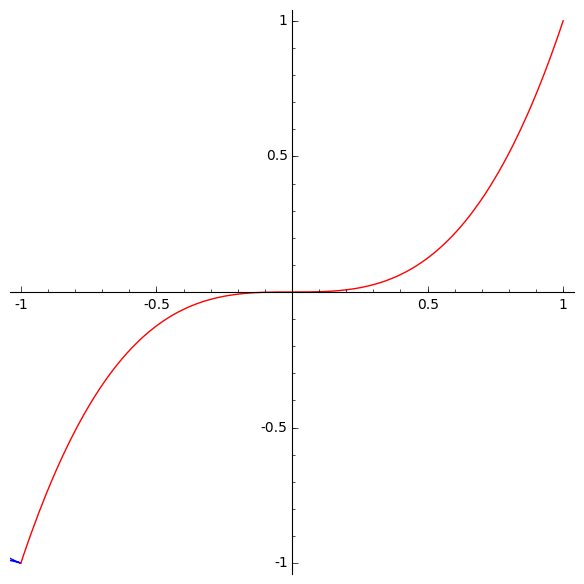The moving blue curve shows all the involutes of the red curve. The involute has a cusp where it hits the line $y = 0$, except when it hits the origin. This cusp should be a cusp of order 5/2. Indeed, you can see that both branches are convex from the same side. But again, the challenge is to prove that these involutes have cusps of order 5/2 where they hit the line $y = 0$. A cusp of order 7/2 would also have two branches that are convex on the same side!

The involutes also have a less pointy cusp where they hit the red curve $y = x^3$. Like the cusp in the tractrix, this should be a cusp of order 3/2, also known as an ordinary cusp.

Marshall Hampton’s animated gif is a beautiful 21st-century version of Arnol’d’s hand-drawn picture:The ‘perestroika’ is the strange thing that happens when the involute crosses the origin.

Posted by: John Baez on March 23, 2016 8:45 PM | Permalink | Reply to this

### Re: The Involute of a Cubical Parabola

Simon Burton made a great animation explaining how one particular involute of the curve $y = x^3$ gets its two cusps: the exciting cusp of order 5/2 where the involute hits the $x$ axis, and the less exciting cusp of order 3/2 where the involute hits the curve $y = x^3$: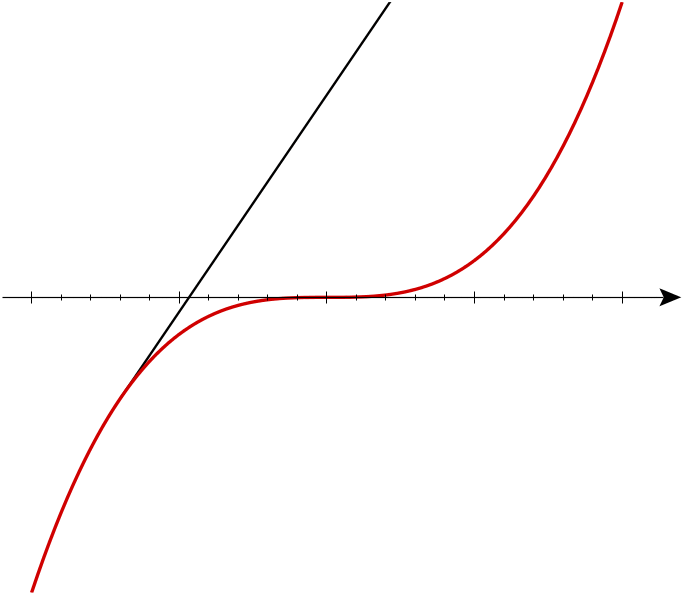Posted by: John Baez on March 23, 2016 11:00 PM | Permalink | Reply to this

### Re: The Involute of a Cubical Parabola

Ok, so I’m stuck in solving the problem. If we parameterize our cubic parabola as $(t, \frac{t^3}{3})$, then using the calculus we have explicit expressions for the involute $D(t) = (x(t), y(t))$, namely

(1)$x = t + \frac{1-s(t)}{\sqrt{1+t^4}}$

and

(2)$y = \frac{t^3}{3} + \frac{(1-s(t)) t^2}{\sqrt{1 + t^4}}$

Here I’ve chosen the “straight line length” of the string to have length 1 at $t=0$, to roughly match with Simon Burton’s animation. Also, $s(t)$ is the arclength of the cubic $y=x^3/3$, namely

(3)$s(t) = \int_0^t \sqrt{1 + u^4} d u.$

So what we have to do analytically is expand these expressions in Taylor series around the cusp point.

But where I get stuck is: how will we recognize a cusp of order $5/2$ in a Taylor series expansion of $x(t)$ and $y(t)$? If we don’t know what we’re looking for, how will we know when we’ve found it…

Posted by: Bruce Bartlett on March 23, 2016 11:24 PM | Permalink | Reply to this

### Re: The Involute of a Cubical Parabola

I’m getting a bit worn out for today, but there are some potentially useful (and certainly interesting) clues on the Wikipedia section Cusp singularity: examples. There they mainly teach us how to recognize different kinds of cusps in curves given by an implicit equation

$f(x,y) = 0$

not a parametric equation.

It’s also worth looking at the section Cusp singularity: classification in differential geometry, because it’s short and it touches on how these cusps show up in Arnol’d’s ADE classification of singularities.

It mentions that there are some subtleties when working with real plane curves: instead of just type $A_k$, when $k$ is even we get two types $A_k^+$ and $A_k^-$, which have as archetypal examples the curves

$x^2 \pm y^{k + 1} = 0$

When $k$ is even these are the same up to a change of coordinates, so we can just say $A_k$.

So, the ‘cusp of order 5/2’ or ‘rhamphoid cusp’ that we seek is a singularity of type $A_4$. This is probably worth remembering when we get deeper into the Dynkin diagram aspects of this story.

Posted by: John Baez on March 23, 2016 11:47 PM | Permalink | Reply to this

### Re: The Involute of a Cubical Parabola

So, anyways, as I was trying to say, relative to the tangent/normal basis (and using $\nu' = -\kappa C'$), we have $C^{(2)} = \kappa \nu$ $C^{(3)} = \kappa' \nu - \kappa^2 C'$ $C^{(4)} = (\kappa''-\kappa^3)\nu - 3\kappa\kappa' C'$ $C^{(5)} = (\kappa^{(3)} - 6\kappa^2\kappa')\nu + (\kappa^4-4\kappa''\kappa - 3 \kappa'^2) C'$ so that whatever involute has $D' = - s C''$ $D'' = - C'' - s C^{(3)}$ $D^{(3)} = -2C^{(3)} - s C^{(4)}$ $D^{(4)} = -3C^{(4)} - s C^{(5)}$ and we are interested in a generic involute where it meets the sinuglar tangent line — w.l.o.g, let’s set $s=1$; the inflection point on the cubic has $\kappa(s=1)=0$ and $\kappa'\neq 0$. So we get $D' = - \kappa \nu = 0$ $D'' = -(\kappa + \kappa')\nu) - \kappa^2 C' = - \kappa'\nu$ $D^{(3)} = (-2\kappa' - \kappa'' - \kappa^3)\nu + (-2\kappa^2 + 3\kappa\kappa') C'$ ${} = -(2\kappa' + \kappa'') \nu$ $D^{(4)} = (-3 \kappa'' + 3 \kappa^3 - \kappa^{(3)} - 6\kappa^2\kappa')\nu + (9\kappa\kappa' - \kappa^4 + 4\kappa''\kappa + 3 \kappa'^2) C'$ ${} = (-3\kappa'' - \kappa^{(3)}) \nu - 3\kappa'^2 C'$ Near the singular point $D(1)$, where $D'=0$, the curve $D(1+t)$ looks like $\left(x_1 + \frac{t^4}{8} \kappa'^2 + \dots, y_1 + \frac{t^2}{2} \kappa' - \frac{t^3}{3} (2\kappa' +\kappa'') + \dots\right)$

So, just for fun, consider the parametric curve $x=t^4, y = t^2 + t^3$ One can exactly eliminate $t$ to get $−x^3−4 x^2 y+x^2−2 y^2 x +y^4 = 0$ but it might be more helpful to formally solve for $t$ as a power series in $u$ where $y = u^2$.

Posted by: Jesse C. McKeown on March 24, 2016 1:25 AM | Permalink | Reply to this

### Re: The Involute of a Cubical Parabola

On second thought, no, don’t solve for $t$ as a power series in whatever; the normalized approximation $(x,y) = ( t^4 , t^2 + t^3 )$ has two branches corresponding to $t ≶ 0$ ; so, suppose $t^2 + t^3 = u^2 + u^3$; which means $t^2 - u^2 = u^3 - t^3$, which means $t^4 - u^4 = (u^3-t^3)(u^2 + t^2)$. Supposing $t$ small means supposing $u = t$ or $u \sim - t$; the latter means $t^4 - u^4 \sim 4 u^5$. That is, if $(x,y_1)$, $(x,y_2)$ are different points near the cusp, we have $x \sim u^2$ and $y_2 - y_1 \sim 4 u^5$; or $(y_2-y_1)^2 \sim 16 x^5$.

Posted by: Jesse C. McKeown on March 24, 2016 9:12 PM | Permalink | Reply to this

### Re: The Involute of a Cubical Parabola

sorry, I seem to have switched $x$ and $y$ near the end.

Posted by: Jesse C. McKeown on March 24, 2016 9:17 PM | Permalink | Reply to this

### Re: The Involute of a Cubical Parabola

I’m having trouble understanding all this, so I’ll start with the obvious question: are you hinting that you’ve solved the problem I posted?

Posted by: John Baez on March 24, 2016 9:16 PM | Permalink | Reply to this

### Re: The Involute of a Cubical Parabola

At least I’m saying that I’ve answered Bruce’s question about Taylor series and a 5/2-cusp: A 5/2-cusp generically arises from a parametric curve with Taylor series $(t^2 + t^3 + \dots, t^4 + \dots)$, but that $(t^2 + t^3 + \dots , t^4 + 2 t^5 + \dots )$ is more singular.

I’ve also listed enough derivatives of $C$ to work out enough derivatives of $D= C - s C'$, given $C$ parametrized by arclength, to show that the leading terms of $D$ are probably of the required form. Admittedly, the listing I’ve given here makes it look rather breathless…

On the other hand, I was already quite happy with Arnol’d mentioning of the inflection point as center of curvature for both branches of the cusp.

Posted by: Jesse C. McKeown on March 24, 2016 10:04 PM | Permalink | Reply to this

### Re: The Involute of a Cubical Parabola

Jesse wrote:

At least I’m saying that I’ve answered Bruce’s question about Taylor series and a 5/2-cusp: A 5/2-cusp generically arises from a parematric curve with Taylor series $(t^2 + t^3 + \dots, t^4 + \dots)$, but that $(t^2 + t^3 + \dots , t^4 + 2 t^5 + \dots )$ is more singular.

So you’re saying you’ve got a general method for proving that certain cusps have order 5/2, but that this method does not apply to the cusp we’re actually looking at?

(I may sound grumpy, but I’m just trying to find the forest in the trees here.)

Jesse wrote:

On the other hand, I was already quite happy with Arnol’d his mentioning of the inflection point as center of curvature for both branches of the cusp.

As I mentioned in my post, a cusp of order 7/2 would also have that property. So, his observation doesn’t by itself prove that we’ve got a cusp of order 5/2… unless I’m missing something, which is entirely possible.

Posted by: John Baez on March 24, 2016 10:35 PM | Permalink | Reply to this

### Re: The Involute of a Cubical Parabola

So you’re saying you’ve got a general method for proving that certain cusps have order 5/2, but that this method does not apply to the cusp we’re actually looking at?

On the contrary! The cusp analysis described is chosen specifically to apply to the Taylor series calculated for the involute $D$ where it meets the tangent to a simple inflection point of $C$.

(I may sound grumpy, but I’m just trying to find the forest in the trees here.)

I frustrate me too; never know what to include, what to leave out as being too obvious, what to leave out for other reasons… and I change my mind about it all the time.

Jesse wrote:

On the other hand, I was already quite happy with Arnol’d his mentioning of the inflection point as center of curvature for both branches of the cusp.

So I did!

As I mentioned in my post, a cusp of order 7/2 would also have that property. So, his observation doesn’t by itself prove that we’ve got a cusp of order 5/2… unless I’m missing something, which is entirely possible.

Yes… quite right, of course; though it would be surprising to find that a simple inflection point gave a less-than-generic self-osculating cusp, on the other hand such surprises are … kind-of what we look for, aren’t they?

Posted by: Jesse C. McKeown on March 25, 2016 5:41 AM | Permalink | Reply to this

### Re: The Involute of a Cubical Parabola

I’d like to pose another related puzzle.

Vladimir Arnol’d claims that the involutes of the cubical parabola:can be seen as slices of this surface in 3d space: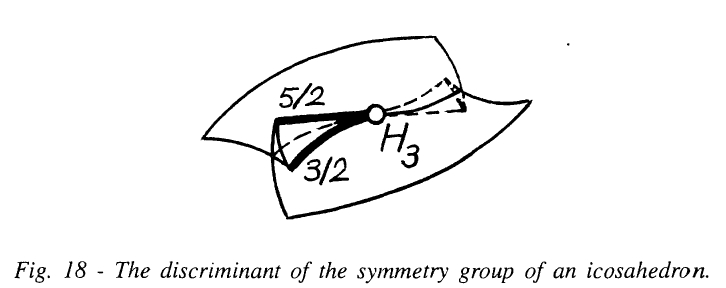after these slices are projected down to a plane.

This surface is “the discriminant of the symmetry group of an icosahedron”. Concretely, up to a coordinate transformation, it’s the surface consisting of points $(a,b,c) \in \mathbb{R}^3$ for which the polynomial

$x^5 + a x^2 + b x^2 + c$

has repeated roots.

We can describe this surface even more concretely by working out the ‘discriminant’ of the above polynomial. This is a function $D(a,b,c)$ that vanishes precisely when $x^5 + ax^2 + b x^2 + c$ has repeated roots.

At this point, over on my other blog, Greg Egan came in and — deus ex machinatold us that

$\begin{array}{ccl} D(a,b,c) & = & c \left(256 a^5 c^2-128 a^4 b^2 c + 16 a^3 b^4+ \right. \\ && \left. 2000 a^2 b c^2-900 a b^3 c+108 b^5+3125 c^3\right) \end{array}$

So, now I’m hoping someone with good computer skills can draw a 3d picture of the surface

$D(a,b,c) = 0$

allowing us to visually compare it to this:You may need to rotate it or warp it a bit to make it look similar.

Posted by: John Baez on March 25, 2016 12:37 AM | Permalink | Reply to this

### Re: The Involute of a Cubical ParabolaThe wiki entry for discriminants has a picture of the discriminant surface for the quartic which looks remarkably similar:Posted by: Archisman Rudra on March 29, 2016 4:40 PM | Permalink | Reply to this

### Re: The Involute of a Cubical Parabola

Thanks, that’s nice!

I did the experiment of posting this blog article both here and on Azimuth. The discussion is mainly occuring there now. In particular, Greg Egan and Simon Burton have created some very nice pictures related to the discriminant of the quintic

$x^5 + a x^4 + b x^2 + c$

to check Arnol’ds claim that Lyashko proved that surface is diffeomorphic to this one:So, I urge everyone interested in this discussion to go there. Later, I will summarize all our results on Visual Insight.

Posted by: John Baez on March 29, 2016 5:34 PM | Permalink | Reply to this

### Re: The Involute of a Cubical Parabola

Yes, it is similar but not the same.

The discriminant surface of the quartic is called the swallowtail. It can be described as the set of points $(a,b,c)$ such that

(1)$x^4 + ax^2 + bx + c$

has multiple roots. The two “sharp points” (i.e. cusp points) in a slice of the picture locally look like $y^2 = x^3$.

The discriminant surface of the symmetry group of an icosahedron (John’s “Fig 18” from Arnold’s book) can be described as the set of points $(a,b,c)$ such that

(2)$x^5 + ax^4 + bx^2 + c$

has multiple roots. The two cusp points in a slice of the picture are different, one locally looks like $y^2 = x^3$ and the other “sharper” one like $y^2 = x^5$.

Posted by: Bruce Bartlett on March 29, 2016 5:59 PM | Permalink | Reply to this

Post a New Comment